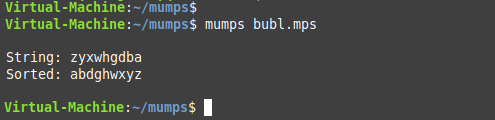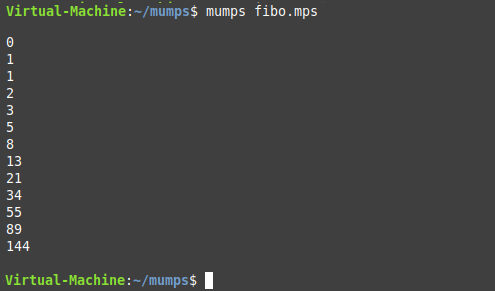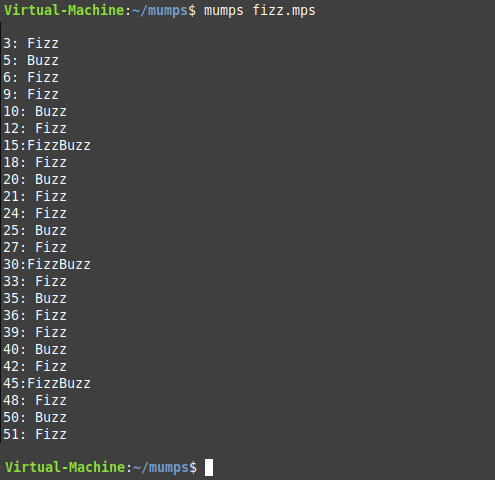# MUMPS Programming Language Snippets

Recently I had cause to learn something about the now somewhat elderly programming language MUMPS ("Massachusetts General Hospital Utility Multi-Programming System"), also sometimes known just as M. It's a very different type of language to modern programming languages, and at first somewhat cryptic. However, with a little persistence, it was intriguing to write a few simple programs, which I have listed here.

While MUMPS may seem like a programming relic from a far different age (it was, in fact, created in 1966, so hardly surprising if it does seem from a different age), there are still systems which use MUMPS-like languages, and derivatives of the original language, so it's really not quite as obsolete as one might imagine. There are also several MUMPS programming resources available online, where you can at least learn the basics, and there exist several distributions of the MUMPS language which you can download and install on your favourite flavour of Linux. (I chose to install it on a Linux Mint installation running in Microsoft's Hyper-V).

#### Some Example MUMPS Code

There were three programs which I chose to create, trying to learn some of the basics. These may possibly not be the most precise ways of accomplishing the tasks, but each of the programs works correctly, producing the desired output.

The first program is a simple bubble-sort, sorting the characters of a string:

```#!/usr/bin/mumps

set string="zyxwhgdba"
w !,"String: ",string,!

for i:1:1 do quit:i>(\$len(string))
. set arr(i)=\$extract(string,i)

for h:1:1 do quit:h>(\$len(string))
. for i:1:1 do quit:'\$data(arr(i+2))
.. set x=i+1
.. set t1=arr(i)
.. set t2=arr(x)

.. if (\$ascii(t1)>\$ascii(t2)) do
... set arr(x)=t1
... set arr(i)=t2

w "Sorted: "

for i:1:1 do quit:'\$data(arr(i+1))
. w arr(i)

w !!```

Output:##### MUMPS - Fibonacci Sequence

This second program prints all the numbers less than a hundred from the Fibonacci Sequence (the Fibonacci Sequence is created by the next number in the sequence being determined by adding up the two numbers previous to it):

```#!/usr/bin/mumps

fibby ; second program
set (a,tot)=0
set b=1
for i=0:1 do quit:tot>100
. if i=0 w !a
. if i=1 w !b
. set tot=a+b
. set a=b,b=tot
. w !,tot

w !!```

Output:#### MUMPS - FizzBuzz

FizzBuzz is a very common programming exercise to demonstrate at least some basic level of familiarity with a language or programming concepts. Being asked to write a FizzBuzz program is the type of question that one might find on an interview for an entry-level programming job. What is FizzBuzz? Okay, it's simply a program which prints a series of numbers. For the numbers which are multiples of 3, the program prints "Fizz", and for numbers which are multiples of 5, the program prints "Buzz". For numbers which are multiples of each (eg. 15), the program prints "FizzBuzz".

```#!/usr/bin/mumps

Fizz ; July 2022

for i=1:1 do quit:i>50
. if (i#3=0)&(i#5=0) w !,i,":FizzBuzz" continue
. if i#3=0 w !,i,": Fizz" continue
. if i#5=0 w !,i,": Buzz"

w !!```

Output:As you might conclude from the code shown above, MUMPS (much like the modern-day Python), unlike many languages, has sensitivity to whitespace, and one needs to code accordingly.

While you may never need to use MUMPS, or anything even remotely like it, it was a bit of fun to challenge myself with quickly learning the basics of a new (to me at least) language.